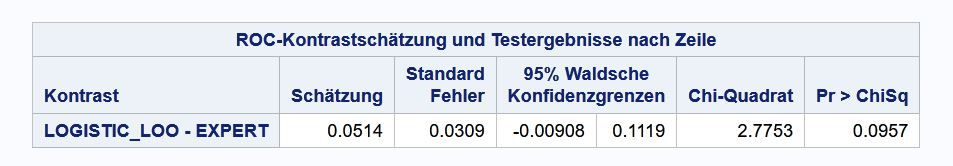## Comparing of two ROC curves, one with manually input

I am currently writing my master thesis with SAS. Therefore, I want to compare two ROC curves within the PROC LOGISTIC Procedure:

One ROC curve will be calculated with the following model:

model DEAL (event='1') =  PRES44_1 SIGNAL3_1 COMCOM4_1;

For the other curve I have a variable with estimated probabilities as a manually input from my study participants. The binary response (dependent variable) is the same for both curves (DEAL).

I like to find out if it is possible to compare both curves regarding the area under the curves?

Best regards,

Joerg

1 ACCEPTED SOLUTION

Accepted Solutions

## Re: Comparing of two ROC curves, one with manually input

Create a data set that contains the observed responses and the predicted probabilities for each model (including the manually generated probabilities). Then you can use the ROC statement in PROC LOGISTIC to create and overlay the ROC curves. The syntax will look like:

``````proc logistic data=Have;
model Y = LogiPred ManualPred / nofit;
roc 'Logistic' pred=LogiPred ;
roc 'Expert'   pred=ManualPred;
ods select ROCCurve ROCOverlay;
run;
``````

For more discussion and an example that shows how to create and overlay ROC curves, see the article "Create and compare ROC curves for any predictive model."

6 REPLIES 6Ksharp
Super User

## Re: Comparing of two ROC curves, one with manually input

Using OUTPUT statement to save probability. and Plot ROC by yourself.

``````
/********Plot ROC curve***********/
options validvarname=any;

data have;
set x.score_card;
run;

proc sort data=have(keep=total_score) out=score nodupkey;
by descending total_score;
run;
data score;
set score end=last;
output;
if last then do;total_score=total_score-1;output;end;
run;
proc sort data=score;
by total_score;
run;

proc sort data=have;
run;

proc delete data=want;run;
%macro roc(score=);
data temp;
set have;
run;
proc sql;
create table temp1 as
from temp
quit;
proc transpose data=temp1 out=temp2;
var percent;
run;
data temp3;
score=&score;
drop _name_;
run;
proc append base=want data=temp3 force;
run;
%mend;

data _null_;
set score;
call execute(cats('%roc(score=',total_score,')'));
run;

data roc;
set want;
dx=-dif(_1_minus_specifity);
dy=mean(sensitity,lag(sensitity));
roc=dx*dy;
run;

proc sql noprint;
select sum(roc) into : roc from roc;
quit;

proc sgplot data=want aspect=1 noautolegend;
lineparm x=0 y=0 slope=1/lineattrs=(color=grey);
series x=_1_minus_specifity y=sensitity;
inset "ROC = &roc"/position=topleft;
xaxis grid;
yaxis grid;
run;
``````

## Re: Comparing of two ROC curves, one with manually input

Create a data set that contains the observed responses and the predicted probabilities for each model (including the manually generated probabilities). Then you can use the ROC statement in PROC LOGISTIC to create and overlay the ROC curves. The syntax will look like:

``````proc logistic data=Have;
model Y = LogiPred ManualPred / nofit;
roc 'Logistic' pred=LogiPred ;
roc 'Expert'   pred=ManualPred;
ods select ROCCurve ROCOverlay;
run;
``````

For more discussion and an example that shows how to create and overlay ROC curves, see the article "Create and compare ROC curves for any predictive model."

## Re: Comparing of two ROC curves, one with manually input

Hi Rick,

Thanks for your help. I did not know the /nofit option.

Now, I can also test the differences between the ROC curves:

proc logistic data=s4y.dealpred plots=roc(id=prob);
model DEAL (event='1') =  PRED_ PROBFORE_PZ / nofit;
roc 'Expert' PROBFORE_PZ;
roc 'Logistic' PRED_;
roccontrast;
run;

Best regards,

Joerg

## Re: Comparing of two ROC curves, one with manually input

Hi Rick,

I am still struggling with my master thesis. There is one question left regarding the comparing of two ROC curves with the DeLong approach. My hypothesis is formulated in way that it needs a one-tailed chi-square-test, meaning I claimed that the difference of the areas between LOGISTIC_LOO and EXPERT is positive:Can I halve the p-value for the described scenario?

Best regards,

Joerg

## Re: Comparing of two ROC curves, one with manually input

Can I halve the p-value for the described scenario?

I don't think so. Chi-square tests are one-sided by their construction.

Your contrast shows that the area under the two ROC curves are not significantly different at the alpha=0.05 significance level. The best you can claim is that they are (barely) different for alpha=0.1, and the estimate shows that LOGISTIC_LOO is greater in area than EXPERT.

Hi Rick,